# JAC Class 10 Maths Solutions Chapter 13 Surface Areas and Volumes Ex 13.3

Jharkhand Board JAC Class 10 Maths Solutions Chapter 13 Surface Areas and Volumes Ex 13.3 Textbook Exercise Questions and Answers.

## JAC Board Class 10 Maths Solutions Chapter 13 Surface Areas and Volumes Exercise 13.3

Take π = $$\frac{22}{7}$$, unless stated otherwise.

Question 1.
A metallic sphere of radius 4.2 cm is melted and recast into the shape of a cylinder of radius 6 cm. Find the height of the cylinder.
Solution:
R = 4.2, r = 6
Volume of the cylinder = Volume of the melted sphere
πr2h = $$\frac{4}{3}$$πR3
3πr2h = 4πR3
h = $$\frac{4 \pi \mathrm{R}^3}{3 \pi \mathrm{r}^2}$$
= $$\frac{4 \times 4.2 \times 4.2 \times 4.2}{3 \times 6 \times 6}$$
= 4 × 1.4 × 0.7 × 0.7
= 5.6 × 0.49
= 2.744 cm.
Height of the cylinder = 2.74 cm.

Question 2.
Metallic spheres of radii 6 cm, 8 cm and 10 cm, respectively, are melted to form a single solid sphere. Find the radius of the resulting sphere.
Solution:
r1 = 6 cm, r2 = 8 cm, r3 = 10 cm.
Let the radius of the resulting sphere be r cm.
Its volume = $$\frac{4}{3}$$πR3
Volume of the resulting sphere = Volume of the sphere of radius r1 + Volume of the sphere of radius r2 + Volume of the sphere of radius r3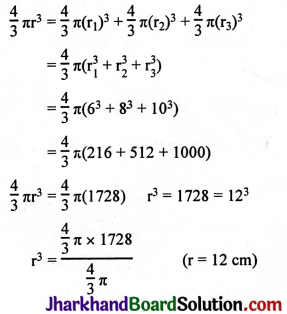Radius of the resulting sphere = 12 cm.Question 3.
A 20 m deep well with diameter 7 m is dug and the earth from digging is evenly spread out to form a platform 22 m by 14 m. Find the height of the platform.
Solution: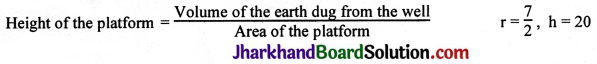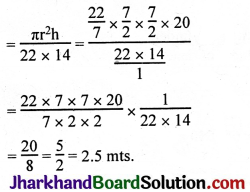Question 4.
A well of diameter 3 m is dug 14 m deep. The earth taken out of it has been spread evenly all around it in the shape of a circular ring of width 4m to form an embankment. Find the height of the embankment.
Solution:
Volume of the earth got by digging the well = πr2h
= $$\frac{22}{7} \times \frac{3}{2} \times \frac{3}{2} \times 14$$
= 99 cm.
R = 1.5 + 4
r = 1.5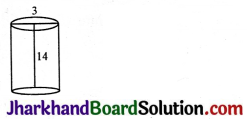Area of the circular embankment around the well = πR2 – πr2
= π[(5.5)2 – (1.5)2]
= π(5.5 + 1.5) (5.5 – 1.5)
= π(7) (4).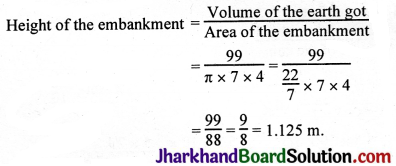Question 5.
A container shaped like a right circular cylinder having diameter 12 cm and height 15 cm is full of ice cream. The ice cream is to be filled into cones of height 12 cm and diameter 6 cm, having a hemispherical shape on the top. Find the number of such cones which can be filled with ice cream.
Solution:
Volume of ice cream in the cylindrical container =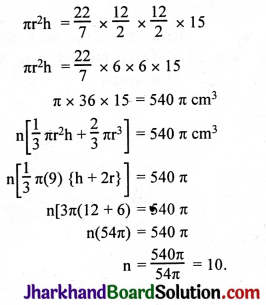Alternative Method:
Number of cones will be = $$\frac{\text { Volume of cylinder }}{\text { Volume of cone }}$$
For the cylinder part,
Radius = $$\frac{12}{2}$$ = 6 cm, Height = 15 cm
∴ Volume of cylinder = π × r2 × h = 540π
For the cone part,
Radius of conical part = $$\frac{6}{2}$$ = 3 cm, Height = 12 cm
Radius of hemispherical part = $$\frac{6}{2}$$ = 3 cm
Now,
Volume of cone = Volume of conical part + Volume of hemispherical part
$$\left(\frac{1}{3}\right)$$ × π × r2 × h + $$\left(\frac{2}{3}\right)$$ × π × r3
= 36π + 18π
= 54π.
∴ Number of cones = $$\frac{540 \pi}{54 \pi}$$ = 10

Question 6.
How many silver coins, 1.75 cm in diameter and of thickness 2 mm, must be melted to form a cuboid of dimensions 5.5 cm × 10 cm × 3.5 cm?
Solution: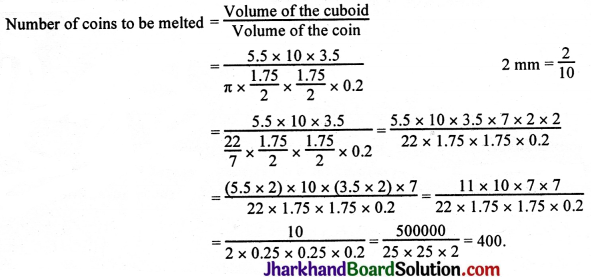Question 7.
A cylindrical bucket, 32 cm high and with radius of base 18 cm, is filled with sand. This bucket is emptied on the ground and a conical heap of sand is formed. If the height of the conical heap is 24 cm, find the radius and slant height of the heap.
Solution: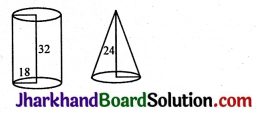Volume of sand in the bucket = πr2h
= π × 18 × 18 × 32 cc.
Volume of the conical heap of sand = $$\frac{1}{3}$$πr2h
$$\frac{1}{3}$$πr2h = π × 18 × 18 × 32
πr2h = 3 × π × 18 × 18 × 32
πr2 × 24 = 3 × π × 18 × 18 × 32
r2 = $$\frac{3 \times \pi \times 18 \times 18 \times 32}{\pi \times 24}$$
r2 = 182 × 22
r = 18 × 2 = $$\frac{72}{2}$$ = 36 cm.
l2 = h2 + r2
= (24)2 + (36)2
= (24 × 24) + (36 × 36)
= (2 × 12 × 12 × 2) + (3 × 12 × 12 × 3)
= 122 (4 + 9)
= 122 × 13
l = 12$$\sqrt{13}$$ cm.

Question 8.
Water in a canal, 6 m wide and 1.5 m deep, is flowing with a speed of 10 km/h. How much area will it irrigate in 30 minutes, if 8 cm of standing water is needed?
Solution:
Quantity of water flowing in the canal in 1 hour = lbh
l = 10 km, b = 6 m, h = 1.5 m
10 × 1000 × 6 × 1.5 cubic metres.
The area this water can irrigate in 1 hour if 8 cm of standing water is needed
= $$\frac{10 \times 1000 \times 6 \times 1.5}{0.08}$$
8 cm = 0.08 mm
Area needed to irrigate in 30 mins. or $$\frac{1}{2}$$ hr.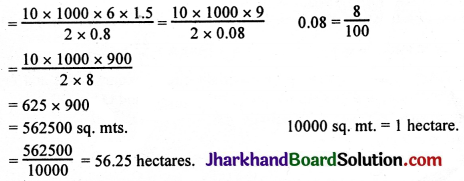Question 9.
A farmer connects a pipe of internal diameter 20 cm from a canal into a cylindrical tank in her field, which is 10 m in diameter and 2 m deep. If water flows through the pipe at the rate of 3 km/h, in how much time will the tank be filled?
Solution:
d = 20 cm, r = 10 cm = $$\frac{10}{100}$$ = 0.1 mt, h = 3 km = 3 × 1000 m.
Volume of water that comes out of the pipe of diameter 20 cm
= πr2h
= π × 0.1 × 0.1 × 3000
= π × 30 cm.
In 1 hr. (60 mins.), volume of water that flows into the tank = π × 30
In 1 minute = $$\frac{\pi \times 30}{60}=\frac{\pi}{2}$$ cm.
Volume of the cylindrical tank = πr2h = π × 5 × 5 × 2. d = 10, r = 5
$$\frac{\pi}{2}$$ cubic metres of water will be filled in 1 minute
π × 5 × 5 × 2……. $$\frac{\pi \times 5 \times 5 \times 2 \times 2}{\pi}$$ = 100 mins.